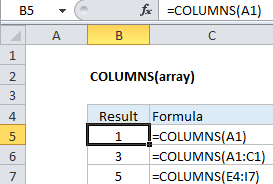How to use Excel COLUMNS Function

This Excel tutorial explains how to use the COLUMNS function with syntax and examples.

Excel COLUMNS function Description

The Microsoft Excel COLUMNS function returns the number of columns in a cell reference.

The COLUMNS function is a built-in function in Excel that is categorized as a Lookup/Reference Function. It can be used as a worksheet function in Excel. As a worksheet function, the COLUMNS function can be entered as part of a formula in a cell of a worksheet.

Also See:   How to count total columns in range in ExcelExplanation: The Excel COLUMNS function returns the count of columns in a given reference. For example, COLUMNS(A1:C3) returns 3, since the range A1:C3 contains 3 columns.

Syntax

The syntax for the COLUMNS function in Microsoft Excel is:

COLUMNS( reference )

Returns

The COLUMNS function returns a numeric value.